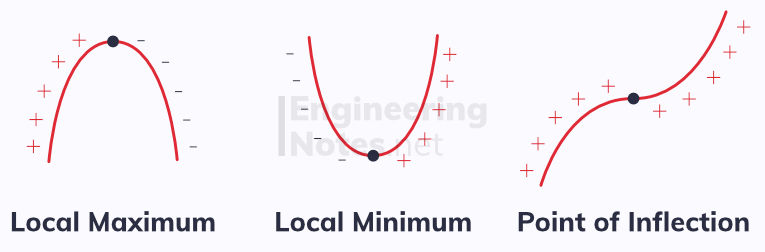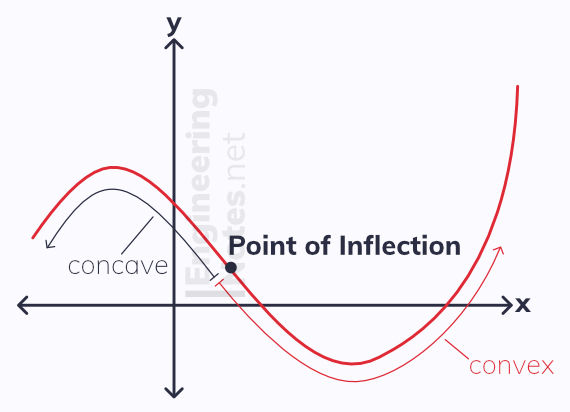top of page

#### Notes by Category University Engineering

Electronics*
Mathematics*
Mechanics & Stress Analysis*
Rate these notesNot a fanNot so goodGoodVery goodBrillRate these notes

# Differentiation

The gradient of a curve is constantly changing, and so finding the gradient is not as simple as reading the x-coefficient of the equation. Instead, we can draw tangents to the curve to calculate the gradient at the point where the tangent touches the curve.The gradient of a curves at a given point is given as the gradient of the tangent to the curve at that point

## Finding the Derivative

However, drawing tangents is not very accurate when done by eye. Instead, we can use algebra to find the gradient of a curve at a given point.

This works by drawing a cord connecting two points on the curve, y = f(x). The gradient of this cord gives an estimate for the average gradient of the curve between the two points. As you can see, the closer the two points are together, the more accurate the gradient of of the cord is as an estimation. This is because the cord gets closer and closer to being parallel to the tangent.This can be noted as a cord between points A and B, where the horizontal distance between the points (difference in x-values) is h. Therefore, the vertical distance (difference in y-values) is f(x₀+h) - f(x₀):Since gradient is defined as change in y-value over change in x-value (rise over run), the gradient of the cord is given as:As the value for h gets smaller, points A and B get closer together, and the gradient of the cord becomes a better estimation for the gradient of the curve at A.

As h → 0, the gradient of the cord is identical to the gradient of the curve at the point.

This is known as differentiation from first principles:### Terminology

• The derivative of a function is the differentiated form of the function

• The derivative of f(x) can be noted as f'(x)

• The derivative of y = f(x) can be noted as dy/dx

• h → 0 means "as h tends to zero" - its value becomes negligible

## Differentiating xⁿ

You do not have to differentiate from first principles every time - it is only used as a proof. Instead, we use general rules to make differentiation quicker. To differentiate xⁿ:

• multiply by the power, n

• reduce the power by one, n becomes n-1

If f(x) = xⁿ, then f'(x) = nxⁿ‾¹
If f(x) = axⁿ, then f'(x) = anxⁿ‾¹
• This applies when n is real, and a is a constant

For functions with more than one term, you differentiate one term at a time, using the rules above. A quadratic is a function where the highest power of x is x², and can be differentiated as such:

If f(x) is ax² + bx + c, then f'(x) = 2ax + b
• This applies when a, b and c are constants and x is the only variable

Note that the derivative is now linear, because all x-terms were reduces by one order. c has disappeared all together, because it can be seen as cx⁰, so when c is multiplied by the power of x, 0, it becomes 0.

### Differentiating Cubics

The exact same rules apply for all functions: differentiate each term individually.

If f(x) = ax³ + bx² + cx + d, then f'(x) = 3ax² + 2bx + c
• This applies when a, b, c and d are constants and x is the only variable

Note that the cubic becomes a quadratic when differentiated.

## Second Order Derivatives

The first order derivative is the rate of change of the function, or the gradient. The second order derivative is the rate of change of the gradient itself, or the rate of change of the rate of change of the function.

It is noted as f''(x) or d²y/dx²

This sounds complicated, but all it means is you differentiate a function twice:

If f(x) = ax³ + bx² + cx + d:

• f'(x) = 3ax² + 2bx + c

• f''(x) = 6ax + 2b

As well as second order derivatives, you can have third, fourth fifth etc order derivatives. However, these are rarely encountered at this stage.

Often, differentiation is used to find the equation of a tangent or normal to a curve at a given point.The tangent to the curve y = f(x) at the point (a, f(a)) has gradient f'(a)
The tangent to the curve y = f(x) at the point (a, f(a)) has equation y - f(a) = f'(a)(x - a)

The normal is the line that is perpendicular to the tangent, and intersects the curve at point A. The gradient of the normal is given as the negative reciprocal of the gradient of the tangent:

The normal to the curve y = f(x) at the point (a, f(a)) has gradient 1 / f'(a)
The normal to the curve y = f(x) at the point (a, f(a)) has equation y - f(a) = -(x - a) / f'(a)
• The negative reciprocal means that the product of the gradients of the tangents and normal equals -1

## Increasing and Decreasing Functions

A function can either be increasing or decreasing over a given interval, depending on whether its derivative is positive or negative.f(x) is increasing on the interval [a, b] if f'(x) ≥ 0 for all values a < x < b
f(x) is decreasing on the interval [a, b] if f'(x) ≤ 0 for all values a < x < b

## Stationary Points

A stationary point is a point on a curve where the gradient is zero. It can take one of three forms:• At a local maximum, the gradient changes from positive to negative

• At a local minimum, the gradient changes from negative to positive

• At a point of inflection, the gradient does not change sign - it can either stay positive or negative throughout.You can determine which type of point it is by looking at what the second order derivative is doing, or by what the first order differential is on either side of the point.

To determine the nature of a stationary point, A:

• If f''(a) > 0, the point is a local minimum

• If f''(a) < 0, the point is a local maximum

• If f''(a) = 0, look at the gradient on either side of A as it could be any of the three## Convex & Concave Curves

All curves are either convex or concave over a particular interval.It is easy to remember which is which, because concave curves look like the entrance to a cave, while convex curves look like a v.A point of inflection is where the curve changes from being concave to being convex or vice versa

To sketch a gradient function of a particular function, this guide applies:

• Maximum or minimum points on f(x) cut the x-axis on f'(x)

• Points of inflection on f(x) touch but do not cross the x-axis on f'(x)

• A positive gradient on f(x) means f'(x) is above the x-axis

• A negative gradient on f(x) means f'(x) is below the x-axis

• Vertical asymptotes on f(x) remain as vertical asymptotes on f'(x)

• Horizontal asymptotes of f(x) become horizontal asymptotes at the x-axis on f'(x)

## The Chain Rule

The chain rule is used to differentiate composite functions:• Where y is a function of u, and u is a function of x

For example, to differentiate y = (3x⁴ + x)⁵: